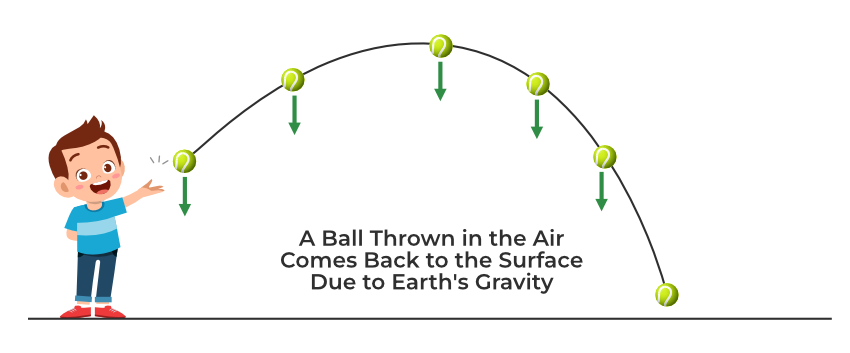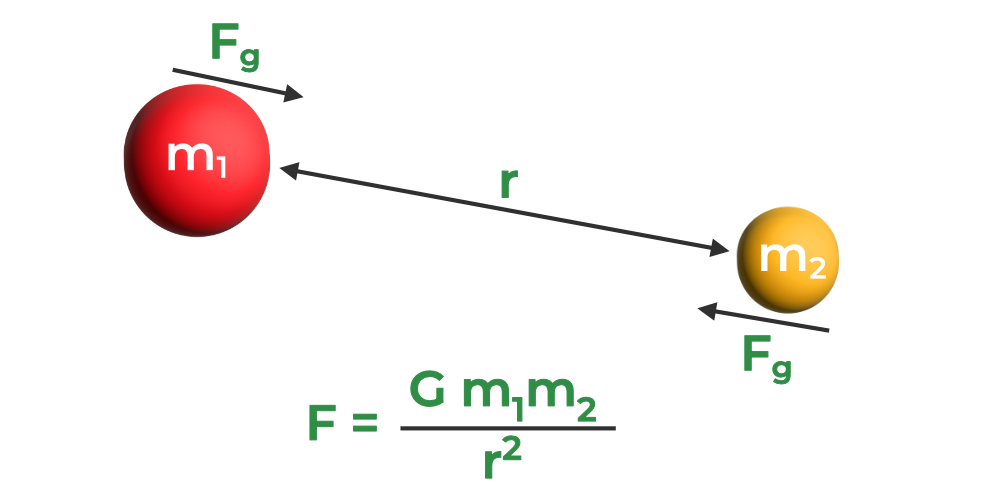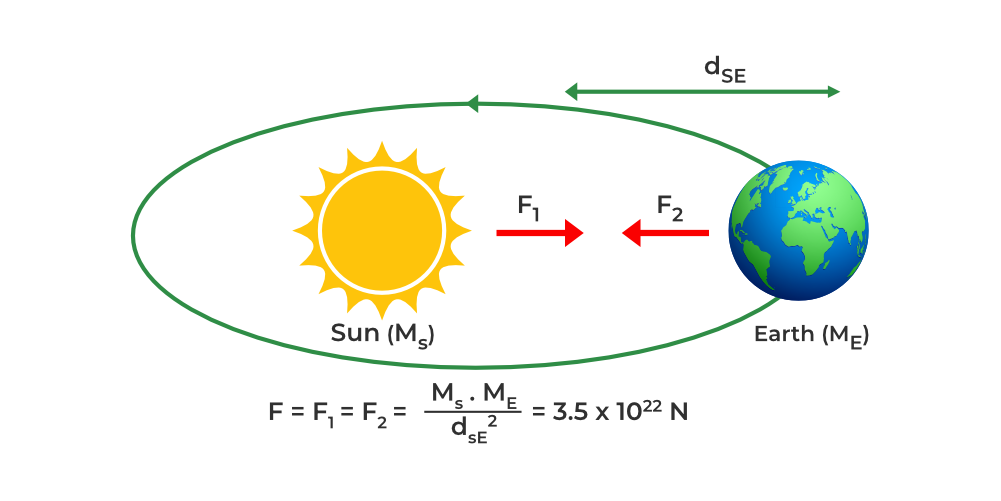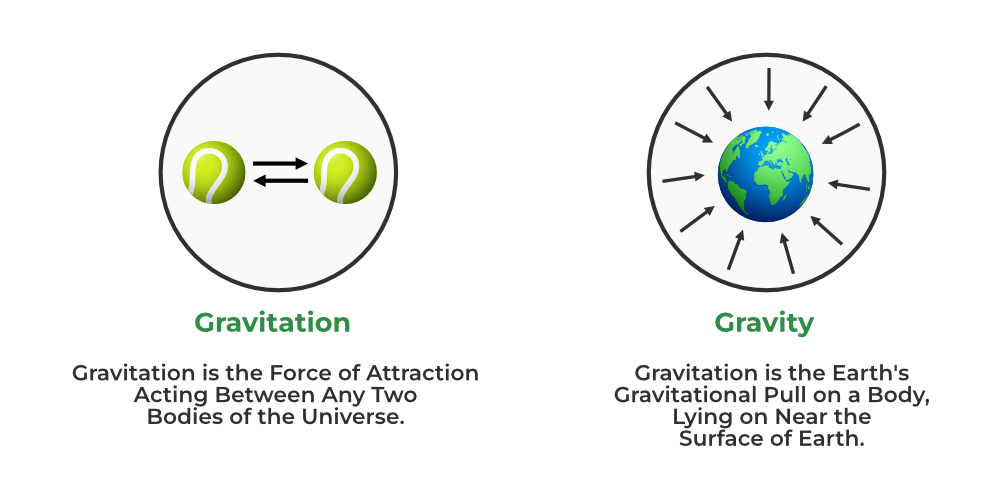GFG App
Open AppBrowser
Continue

Newton’s Law of Universal Gravitation is used to explain gravitational force. Gravitational Force is a type of Non-contact force, the gravitational force is a force in nature that is always attractive and conservative. Gravitational Force is defined as the force of attraction experienced by two or more objects in contact. Gravitational force is determined by the formula obtained from Newton’s Universal Law of Attraction, known as the Gravitational Force Formula. Our environment is surrounded by gravity. It determines how much we weigh and how far a basketball will bounce before hitting the ground when it is thrown. The force the Earth applies to you is equal to the gravitational force on Earth. The gravitational force is equal to your weight while you are at rest on or near the Earth’s surface. Also, the presence of a gravitational field is required in the heat transfer through natural convection.## What is Gravitational Force?

The gravitational force is a force that attracts any two objects in the universe, whether they have equal masses or not. Furthermore, Newton’s Universal Law of Gravitation states that everything, including you, pulls every other object in the universe. The unit of gravitational force is Newtons, denoted as N.

The study of gravitation has benefited a lot from the contributions of many well-known scientists. Early in the 17th century, Italian astronomer Galileo Galilei discovered that all objects accelerate uniformly toward the center of the Earth. In his groundbreaking study from 1687, English mathematician Isaac Newton made the first discovery of gravitational laws.

Gravitation is considered a fundamental force as its influence on any object can be observed readily. Thus, Gravitational force acts on every object that has mass. So gravitational force is a fundamental force. Since there is no touch between the objects, the gravitational force is non-contact. Since it is focused at the center of the orbit in which the object moves, it is centripetal. It is responsible for keeping the body’s orbit. A tug that is directed away from the center is experienced by the rotating body. The centrifugal force is what causes this pulling. Of all the basic forces, the gravitational force is the weakest.

## Newton’s Law of GravitationNewton’s Law of Gravitation or Newton’s Law of Universal Gravitation (or Universal Laws of Gravitation) is the Law that leads to the further study of Gravitation and states that all the objects in the universe having any masses always attract each other with a force of attraction. This force of attraction is called the Gravitational Force (F) which is,

• Directly proportional to the product of the masses (m1 and m2) of the two objects in contact with other, and
• Inversely proportional to the square of the distance (r) between their centers.

The expression or the relation for the above-stated law is given by the gravitational force formula, discussed below:

## Gravitational Force Formula

The Law of Gravitation gives the Gravitational Force (F) between two bodies of masses (m1 and m2) at a distance r, apart from their centers, is given as:

F ∝ m1m2

and

F ∝ 1/r2

Now, combining the above two relations as,

F ∝ m1m2 / r2

or

F = Gm1m2 / r2

where G is the proportionality constant known as Gravitational Constant (= 6.67 ×10−11N⋅ m2/kg2).

## Unit of Gravitational Force

• The SI unit of Gravitational Force is Newton (N).
• The Dimensional Formula of Gravitational Force is [M1L1T-2].

## Properties of Gravitational Force

Here are some important characteristic features of Gravitational Force,

• Gravitational forces are always attractive and the weakest of all the fundamental forces.
• It is a type of Non-Contact Force, as it does not require any physical contact or touch to be experienced by a system of objects.
• Gravitational Force is a Long-range force and does not require any medium.
• The Gravitational Force value at the surface of the Earth is constant.

Also Read: Contact and Non-Contact Forces

## Gravitational Force Examples

Some everyday life examples of gravitational force can be discussed as,

### Gravitational Force of Earth

Every object is subject to the gravitational pull of Earth, a phenomenon known as gravity. We cannot freely float in the air because of gravity, which keeps us on the ground. The force that the Earth and we both apply to the planet is equal. The Earth, however, remains unaffected because of its immense size. If a hung object is let go, it will fall naturally in the direction of the Earth’s center.

### Gravitational Force Between Earth and Moon

Due to the gravitational pull of the Earth and the Moon, the Moon revolves around the Earth. To compute this force, we put their masses and the separation between their two centers into the gravitational force formula. Then, the gravitational force between the earth and the moon was found to be 2 × 1020 N.

### Gravitational Force of the Sun

Because of its massive mass, the Sun exerts a gravitational force whose range is extremely wide. This attracting force causes all planets to orbit the Sun in an elliptical shape. The gravitational force formula can be used to determine the gravitational force acting on Earth from the Sun and was found to be 3.5 × 1022 N.## Difference between Gravity and Gravitational ForceLet’s discuss the key differences between gravity and gravitational force in detail as mentioned in the table below:

## Solved Examples on Gravitational Force

Example 1: Find the gravitational force of attraction between two elephants, one of mass 1000 kg and the other of mass 800 kg, if the distance between them is 5 m.

Solution:

Given: m1 = 1000 kg, m2 = 800 kg, r = 5 m

The formula for gravitational force is given as: FgHere, G =  6.67 ×10−11N⋅ m2/kg2

Substituting the values in the formula, we have:

FgFg = 2.1 × 10-6 N

Example 2: Find the gravitational force of attraction between a man of mass of 50 kg and a bus of mass 1500 kg, if the distance between them is 10 m.

Solution:

Given: m1 = 50 kg, m2 = 1500 kg, r = 10 m

The formula for gravitational force is given as: FgHere, G =  6.67 ×10−11N⋅ m2/kg2

Substituting the values in the formula, we have:

FgFg = 5.0025 × 10-8 N

Example 3: Suppose the gravitational force between two bodies at a certain distance is 4 N. Find the force of attraction if the distance between them is doubled.

Solution:

Newton’s law of gravitation states that the gravitational force between two point like objects is directly proportional to the product of their masses and inversely proportional to the square of the distance between them.

FgThis equation shows that ,for given masses, if r is replaced by 2r, the force becomes 1/4th original force. Therefore ,force of attraction will become 4/4 =1 N.

Example 4: The mass of the Earth is 6 × 1024 kg. The distance between the Earth and the Sun is 1.5 × 1011m. If the gravitational force between the two is 3.5 × 1022N, what is the mass of the Sun?

Solution:

Given: me = 6 × 1024 kg, r = 1.5 × 1011 m and F = 3.5 × 1022 N

The formula for gravitational force is given as: Fg.

⇒ 3.5 × 1022 N =⇒ Mass of sun == 1.967 × 1030 kg

## FAQs on Gravitational Force

Question 1: Define Gravitational Force.

The gravitational force is a force that attracts any two objects in the universe, whether they have equal masses or not. Furthermore, Newton’s Universal Law of Gravitation states that everything, including you, pulls every other object in the universe.

Question 2: What is the Gravitational Force between two objects?

The Gravitational force between the two objects of masses m1 and m2 at a distance r apart from each other can be calculated by using the formula mentioned below:

F = Gm1m2 / r2

where F is the Gravitational Force, and G is the Gravitational Constant.

Question 3: Who discovered Gravitational Force?

English mathematician Isaac Newton made the first discovery of gravitational laws and forces.

Question 4: Write any two Properties of Gravitational Force.

The following are the important properties of gravitational forces:

• Gravitational forces are always attractive and the weakest of all the fundamental forces.
• It is a type of Non-Contact Force, as it does not require any physical contact or touch to be experienced by a system of objects.

Question 5: Explain Gravitational Force by giving a suitable example.

Every object is subject to the gravitational pull of Earth, a phenomenon known as gravity. We cannot freely float in the air because of gravity, which keeps us on the ground. The force that the Earth and we both apply to the planet is equal. The Earth, however, remains unaffected because of its immense size. If a hung object is let go, it will fall naturally in the direction of the Earth’s center.

Question 6: What is the magnitude of the Gravitational Force?